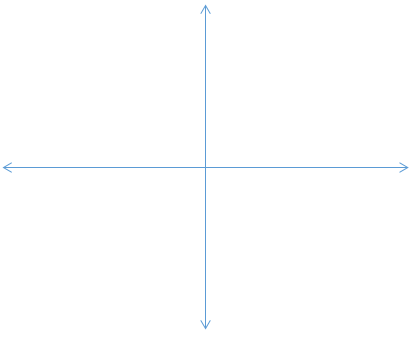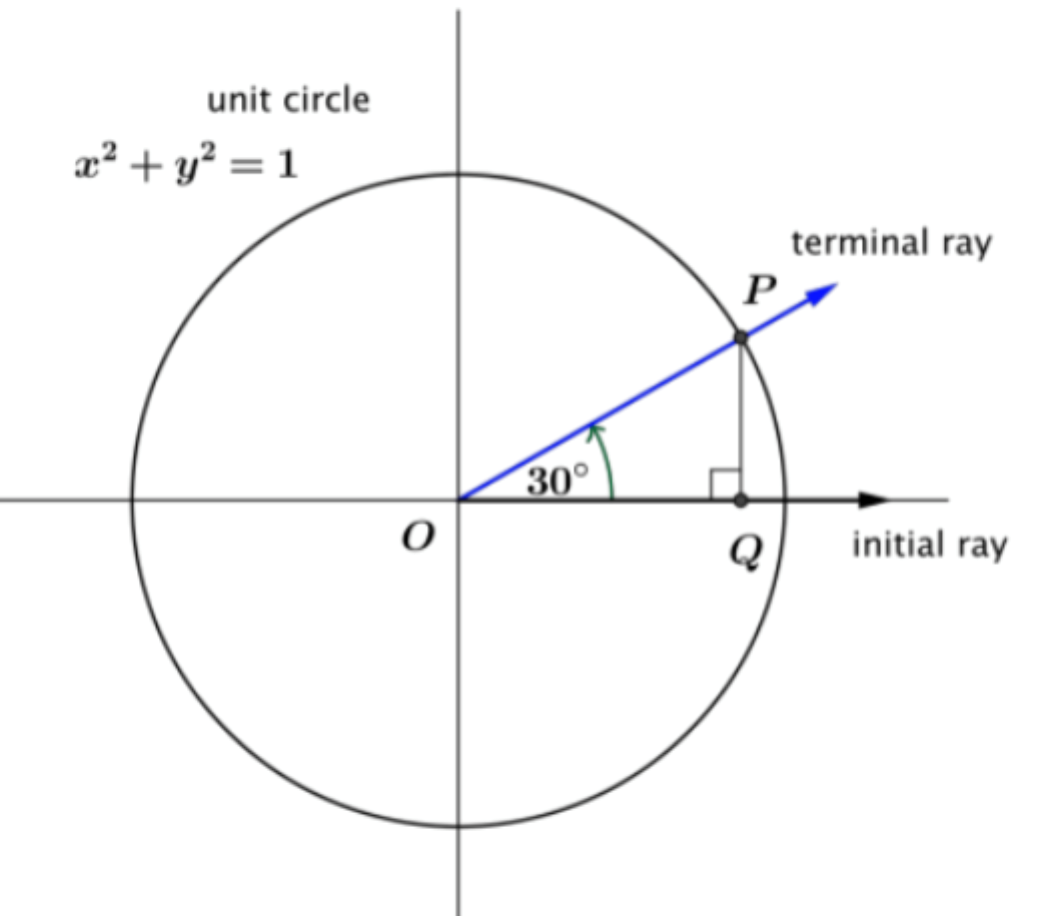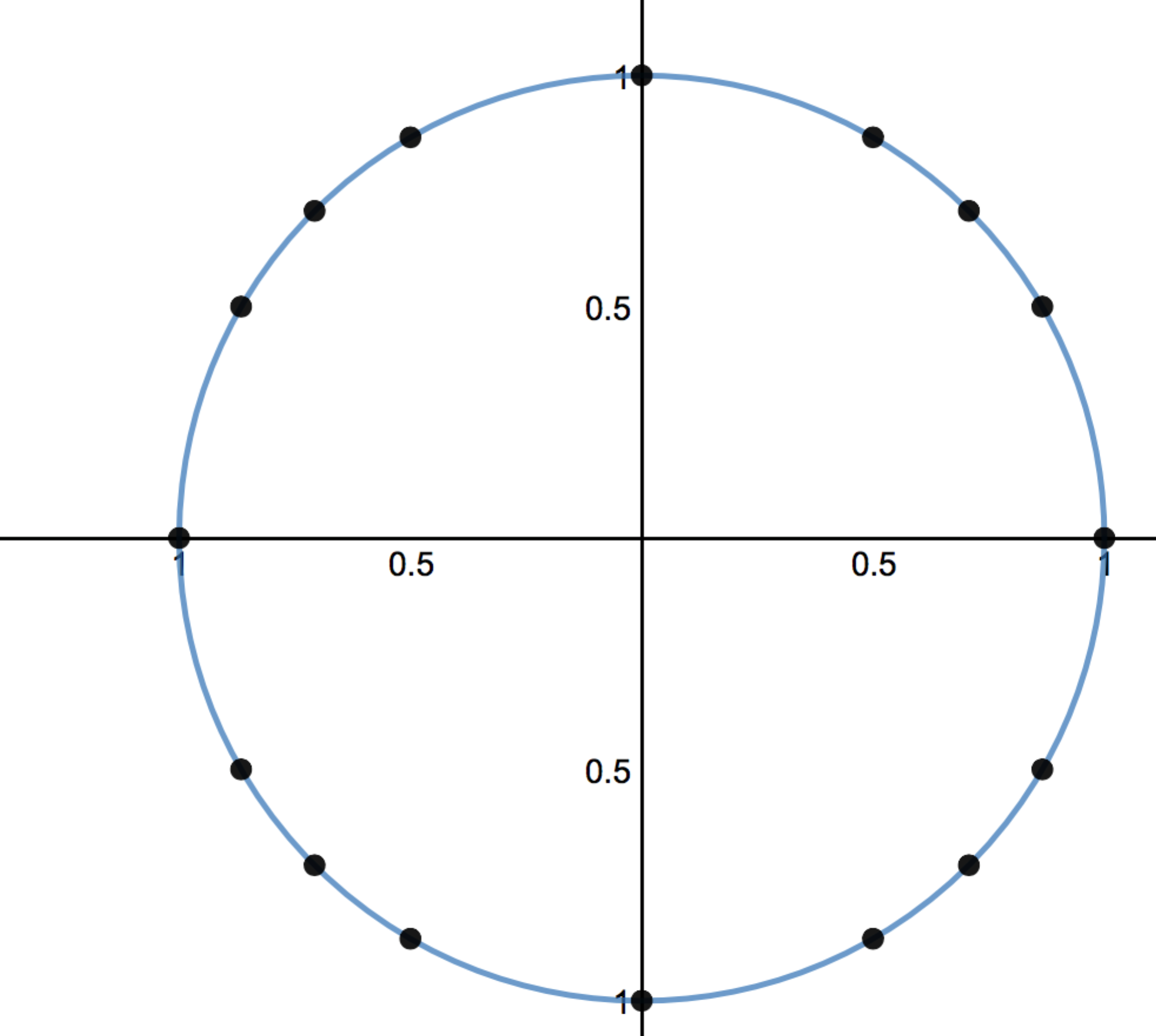# Right Triangles and Trigonometry

## Objective

Define angles in standard position and use them to build the first quadrant of the unit circle.

## Common Core Standards

### Core Standards

?

• F.TF.A.2 — Explain how the unit circle in the coordinate plane enables the extension of trigonometric functions to all real numbers, interpreted as radian measures of angles traversed counterclockwise around the unit circle.

?

• 4.G.A.1

## Criteria for Success

?

1. Identify an angle in standard position if the vertex is at the origin and one ray is on the positive $x$-axis.
2. Orient the initial side with the ray on the $x$-axis and the terminal side as the other ray.
3. Describe the unit circle as a circle where the radii are one unit and each radius is the hypotenuse of triangles formed inside the unit circle.
4. Describe the importance of the unit circle as it relates to trigonometric ratios. This unit circle will be used throughout higher math.
5. Define reference angles as positive and only in the first quadrant.
6. Know that $(x_\theta,y_\theta)=(\mathrm{cos}\theta,\mathrm{sin}\theta)$

## Tips for Teachers

?

• This lesson and the next lesson cover key concepts in the unit by reinforcing and reviewing G-SRT.6 and G-SRT.7.
• The unit circle is used throughout calculus and is built on trigonometric ratios.

## Anchor Problems

?

### Problem 1

Given a blank coordinate plane, draw a 45° angle with a vertex at the origin.### Problem 2

Suppose that point $P$ is the point on the unit circle obtained by rotating the initial ray 30°. Find ${\mathrm{sin}(30^\circ)}$ and ${\mathrm{cos}(30^\circ)}$#### References

EngageNY Mathematics Algebra II > Module 2 > Topic A > Lesson 4Example 1

Algebra II > Module 2 > Topic A > Lesson 4 of the New York State Common Core Mathematics Curriculum from EngageNY and Great Minds. © 2015 Great Minds. Licensed by EngageNY of the New York State Education Department under the CC BY-NC-SA 3.0 US license. Accessed Dec. 2, 2016, 5:15 p.m..

Modified by Fishtank Learning, Inc.

### Problem 3

Each of the points in Quadrant I represent THE point of intersection of a ray that forms a 30° angle, a 45° angle, and a 60° angle with the $x$-axis in the initial position. What are the coordinate points where the rays intersect the circle at 30°, 45°, and 60°?#### References

Unit Circle with Reference Triangles by Match Foundation, Inc. is made available by Desmos. Copyright © 2017 Desmos, Inc. Accessed March 13, 2017, 4:27 p.m..

## Problem Set

?

The following resources include problems and activities aligned to the objective of the lesson that can be used to create your own problem set.

• Include problems where students are asked to identify and fix the error. For example, give the sine of an angle and a drawing on the unit circle with the angle not in standard position.
• Include problems that require students to find the coordinate point based on the angle provided. For example, given an angle, find the coordinate point. Make sure to vary the information given and what is requested for an answer.
• Include problems where students need to make a drawing based on the parameters provided. For example, “Draw an angle that has a sine of… Is there only one angle that will give this sine?”

Draw an angle in standard position that has an intersection point on the unit circle of ${\left(\frac{1}{2},\frac{\sqrt3}{2}\right)}$. What is the sine of this angle? The cosine?